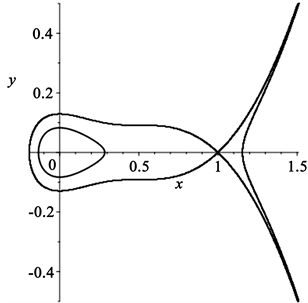# 一类Liénard系统的零点个数最小上界研究A Study on the Upper Number of Zeros for a Liénard System

DOI: 10.12677/PM.2021.117162, PDF, HTML, XML, 下载: 22  浏览: 71

Abstract: In this paper, we aim to use Chebyshev theory of Abel integral generator and polynomial symbolic computing technology to study and analyze the upper bound of the number of zeros of Abel integral corresponding to (4, 3) Liénard system. The minimum upper bound of the number of zeros for I(h,δ) is proved. The number of zeros of Abel integral of following Liénard system is considered. Through the in-depth study of Abel integral I(h,δ), it is proved that the generator of Abel integral can form Chebeyshev system, and a conclu-sion is shown.

1. 背景介绍

$\left\{\begin{array}{l}\frac{\text{d}x}{\text{d}t}={P}_{n}\left(x,y\right)\hfill \\ \frac{\text{d}y}{\text{d}t}={Q}_{n}\left(x,y\right)\hfill \end{array}$

$\stackrel{˙}{x}={H}_{y}\left(x,y\right),\text{ }\stackrel{˙}{y}=-{H}_{x}\left(x,y\right)$

2. 预备知识

1) 若任意的线性组合 ${k}_{0}{f}_{0}\left(x\right)+{k}_{1}{f}_{1}\left(x\right)+\cdots +{k}_{n-1}{f}_{n-1}\left(x\right)$ 在J上最多具有 $n-1$ 个孤立零点，则 $\left\{{f}_{0}\left(x\right),{f}_{1}\left(x\right),\cdots ,{f}_{n-1}\left(x\right)\right\}$ 称为Chebyshev系统(简称T系统)。

2) 若任意线性组合 ${k}_{0}{f}_{0}\left(x\right)+{k}_{1}{f}_{1}\left(x\right)+\cdots +{k}_{n-1}{f}_{n-1}\left(x\right)$，对于 $i=1,2,\cdots ,n$ 最多具有 $i-1$ 个零点(考虑重数)，则 $\left\{{f}_{0}\left(x\right),{f}_{1}\left(x\right),\cdots ,{f}_{n-1}\left(x\right)\right\}$ 称为扩充完全Chebyshev系统(简称ECT系统)。

3) 记 $W\left[{f}_{0}\left(x\right),{f}_{1}\left(x\right),\cdots ,{f}_{k-1}\left(x\right)\right]$ 为如下行列式：

$W\left[{f}_{0}\left(x\right),{f}_{1}\left(x\right),\cdots ,{f}_{k-1}\left(x\right)\right]=\mathrm{det}{\left({f}_{i}^{j}\right)}_{0\le ij\le i-1}=|\begin{array}{cccc}{f}_{0}\left(x\right)& {f}_{1}\left(x\right)& \cdots & {f}_{n-1}\\ {{f}^{\prime }}_{0}\left(x\right)& {{f}^{\prime }}_{1}\left(x\right)& \cdots & {{f}^{\prime }}_{n-1}\left(x\right)\\ ⋮& ⋮& \ddots & ⋮\\ {f}_{0}^{\left(n-1\right)}\left(x\right)& {f}_{1}^{\left(n-1\right)}\left(x\right)& \cdots & {f}_{n-1}^{\left(n-1\right)}\left(x\right)\end{array}|$

${l}_{i}\left(x\right):=\frac{{f}_{i}\left(x\right)}{{A}^{\prime }\left(x\right)}-\frac{{f}_{i}\left(z\left(x\right)\right)}{{A}^{\prime }\left( x \right)}$

1) 当 $i=0,1,\cdots ,n-2$ 时， $W\left[{l}_{0},{l}_{1},\cdots ,{l}_{i}\right]$$\left({x}_{l},{x}_{r}\right)$ 上存在，

2) $W\left[{l}_{0},{l}_{1},\cdots ,{l}_{i}\right]$$\left({x}_{l},{x}_{r}\right)$ 上有零点，用多重数计数，

3) $s>n+k-2$

${\oint }_{{\Gamma }_{h}}F\left(x\right){y}^{k-2}\text{d}x={\oint }_{{\Gamma }_{h}}G\left(x\right){y}^{k}\text{d}x,\text{}\forall k\in N$.

$8{h}^{3}{I}_{i}\left(h\right)={\oint }_{{\Gamma }_{h}}{f}_{i}\left(x\right){y}^{7}\text{d}x\equiv {\stackrel{˜}{I}}_{i}\left( h \right)$

$\left\{{I}_{0}\left(h\right),{I}_{1}\left(h\right),{I}_{2}\left(h\right),{I}_{3}\left(h\right)\right\}$ 是一个ECT系统，并且当且仅当 $\left\{{\stackrel{˜}{I}}_{0}\left(h\right),{\stackrel{˜}{I}}_{1}\left(h\right),{\stackrel{˜}{I}}_{3}\left(h\right)\right\}$ 也是一个ECT系统。

3. 证明过程

$\stackrel{˙}{x}=y,\text{}\stackrel{˙}{y}=x\left(x-1\right){\left(x-\frac{1}{2}\right)}^{2}+\epsilon \left({a}_{0}+{a}_{1}x+{a}_{2}{x}^{2}+{x}^{3}\right)y,\text{}0<\epsilon \ll 1.$

1977年，阿诺德(Arnold)提出弱化的希尔伯特(Hilbert)的第十六问题，目的是研究阿贝尔积分的零点数目

$I\left(h,\delta \right)={\oint }_{{\Gamma }_{h}}q\text{d}x-p\text{d}y,\text{ }h\in J$ (1)

$\stackrel{˙}{x}={H}_{y}\left(x,y\right)+\epsilon p\left(x,y\right),\text{ }\stackrel{˙}{y}=-{H}_{x}\left(x,y\right)+\epsilon q\left(x,y\right),$ (2)

$\stackrel{˙}{x}={H}_{y}\left(x,y\right),\text{ }\stackrel{˙}{y}=-{H}_{x}\left(x,y\right),$ (3)

$\stackrel{˙}{x}=y,\text{ }\stackrel{˙}{y}=\epsilon f\left(x\right)y+g\left(x\right),$ (4)

$\stackrel{˙}{x}=y,\text{}\stackrel{˙}{y}=x\left(x-1\right){\left(x-\frac{1}{2}\right)}^{2}+\epsilon \left({a}_{0}+{a}_{1}x+{a}_{2}{x}^{2}+{x}^{3}\right)y.$ (5)

$H\left(x,y\right)=\frac{1}{2}{y}^{2}-\frac{1}{5}{x}^{5}+\frac{1}{2}{x}^{4}-\frac{5}{12}{x}^{3}+\frac{1}{8}{x}^{2}=\frac{1}{2}{y}^{2}+A\left(x\right),$ (6)

$\left\{{\Gamma }_{h}\right\}\subset \left\{\left(x,y\right)|H\left(x,y\right)=h,\text{}{h}_{0}

${\Gamma }_{h}$ 上的Melnikov函数为：

$I\left(h,\delta \right)={\oint }_{{\Gamma }_{h}}\left({a}_{0}+{a}_{1}x+{a}_{2}{x}^{2}+{x}^{3}\right)\text{d}x\equiv {a}_{0}{I}_{0}\left(h\right)+{a}_{1}{I}_{1}\left(h\right)+{a}_{2}{I}_{2}\left(h\right)+{I}_{3}\left(h\right).$ (7)Figure 1. The portrait of system (5) when ε = 0.

$I\left(h\right)=\frac{1}{2h}{\oint }_{{\Gamma }_{h}}\left(2A\left(x\right)+{y}^{2}\right){x}^{i}y\text{d}x=\frac{1}{2h}\left({\oint }_{{\Gamma }_{h}}2A\left(x\right)y\text{d}x+{\oint }_{{\Gamma }_{h}}{x}^{i}{y}^{3}\text{d}x\right),$ (8)

${\oint }_{{\Gamma }_{h}}2{x}^{i}A\left(x\right)y\text{d}x={\oint }_{{\Gamma }_{h}}{G}_{i}\left(x\right){y}^{3}\text{d}x,$ (9)

$\begin{array}{c}{G}_{i}\left(x\right)=\frac{1}{3}{\left(\frac{2{x}^{i}A\left(x\right)}{A\left(x\right)}\right)}^{\prime }\left(x\right)\\ =\frac{\left(48i{x}^{5}-192i{x}^{4}+48{x}^{5}+304i{x}^{3}-168{x}^{4}-240i{x}^{2}+236{x}^{3}+95ix-170{x}^{2}-15i+70x-15\right){x}^{i}}{45{\left(-1+2x\right)}^{3}{\left(x-1\right)}^{2}}\end{array}$

$\begin{array}{c}{J}_{i}\left(h\right)=\frac{1}{2h}{\oint }_{{\Gamma }_{h}}\left({G}_{i}\left(x\right)+{x}^{i}\right){y}^{3}\text{d}x=\frac{1}{4{h}^{2}}{\oint }_{{\Gamma }_{h}}\left(2A\left(x\right)+{y}^{2}\right)\left({G}_{i}\left(x\right)+{x}^{i}\right){y}^{5}\text{d}\varphi \\ =\frac{1}{4{h}^{2}}{\oint }_{{\Gamma }_{h}}2A\left(x\right)\left({x}^{i}+{G}_{i}\left(x\right)\right){y}^{3}\text{d}x+\frac{1}{4{h}^{2}}{\oint }_{{\Gamma }_{h}}\left({x}^{i}+{G}_{i}\left(x\right)\right){y}^{5}\text{d}x.\end{array}$ (10)

${\oint }_{{\Gamma }_{h}}2A\left(x\right)\left({x}^{i}+{G}_{i}\left(x\right)\right){y}^{3}\text{d}x\text{=}{\oint }_{{\Gamma }_{h}}{E}_{i}\left(x\right){y}^{5}\text{d}x.$ (11)

$\begin{array}{c}{r}_{i}\left(x\right)=2304{i}^{2}{x}^{10}-18432{i}^{2}{x}^{9}+66408{i}^{2}{x}^{8}-163008i{x}^{9}+19584{x}^{10}-139776{i}^{2}{x}^{2}+541920i{x}^{3}\\ -137088{x}^{4}+193696{i}^{2}{x}^{5}-1060176i{x}^{7}+428832{x}^{8}-183840{i}^{2}{x}^{5}+1353896i{x}^{6}-790848{x}^{7}\\ +121120{i}^{2}{x}^{4}-1182420i{x}^{5}+955848{x}^{6}-54720{i}^{2}{x}^{3}+718010i{x}^{4}-796200{x}^{5}+16225{i}^{2}{x}^{2}\\ -301035i{x}^{3}+467270{x}^{4}-2850{i}^{2}x+84050i{x}^{2}-193220{x}^{3}+225i{\text{}}^{2}-14250ix+54825{x}^{2}\\ +1125i-9900x+900.\end{array}$

$\begin{array}{c}{J}_{i}\left(h\right)=\frac{1}{4{h}^{2}}{\oint }_{{\Gamma }_{h}}\left({E}_{i}\left(x\right)+{G}_{i}\left(x\right)+{x}^{i}\right){y}^{5}\text{d}x=\frac{1}{8{h}^{3}}{\oint }_{{\Gamma }_{h}}\left(2A\left(x\right)+{y}^{2}\right)\left({E}_{i}\left(x\right)+{G}_{i}\left(x\right)+{x}^{i}\right){y}^{5}\text{d}\\ =\frac{1}{8{h}^{3}}{\oint }_{{\Gamma }_{h}}2A\left(x\right)\left({E}_{i}\left(x\right)+{G}_{i}\left(x\right)+{x}^{i}\right){y}^{5}\text{d}x+\frac{1}{8{h}^{3}}{\oint }_{{\Gamma }_{h}}\left({E}_{i}\left(x\right)+{G}_{i}\left(x\right)+{x}^{i}\right){y}^{7}\text{d}x.\end{array}$ (12)

${\oint }_{{\Gamma }_{h}}2A\left(x\right)\left({E}_{i}\left(x\right)+{G}_{i}\left(x\right)+{x}^{i}\right){y}^{5}\text{d}x={\oint }_{{\Gamma }_{h}}{D}_{i}\left(x\right){y}^{7}\text{d}x.$ (13)

${D}_{i}\left(x\right)=\frac{1}{7}{\left(\frac{2A\left(x\right)\left({x}^{i}+{G}_{i}\left(x\right)+{E}_{i}\left(x\right)}{A\left(x\right)}\right)}^{\prime }\left(x\right)=\frac{1}{354375{\left(-1+2x\right)}^{9}{\left(x-1\right)}^{6}}{x}^{i}{\stackrel{¯}{g}}_{i}\left(x\right)$，其中

$\begin{array}{c}{\stackrel{¯}{g}}_{i}\left(x\right)=110592{i}^{3}{x}^{15}-1327104{i}^{3}{x}^{14}+4478976{i}^{2}{x}^{15}+7409664{i}^{3}{x}^{13}-51342336{i}^{2}{x}^{14}+57701376i{x}^{15}\\ -25546752{i}^{3}{x}^{12}+273341952{i}^{2}{x}^{13}-632530944i{x}^{14}+234772992{x}^{15}+60855552i{x}^{11}\\ -896894208{i}^{2}{x}^{12}+3214916352i{x}^{13}-2465116416{x}^{14}-106142976{i}^{3}{x}^{10}+2029183488{i}^{2}{x}^{11}\\ -10052225280i{x}^{12}+11981620224{x}^{13}+140091904{i}^{3}{x}^{9}-3354500736{i}^{2}{x}^{10}+21630292992i{x}^{11}\end{array}$

$\begin{array}{c}-35763372288{x}^{12}-142525440{i}^{3}{x}^{8}+4187744064{i}^{2}{x}^{9}-33942149568i{x}^{10}+73328718528{x}^{11}\\ +112724880{i}^{3}{x}^{7}-4022191200{i}^{2}{x}^{8}+40144961792i{x}^{9}-109441242720{x}^{10}-69325920{i}^{3}{x}^{6}\\ +2998330200{i}^{2}{x}^{7}-36465079440i{x}^{8}+122889085920{x}^{9}+32887200{i}^{3}{x}^{5}-1735866300{i}^{2}{x}^{6}\\ +25667156060i{x}^{7}-105792127920{x}^{8}-11818800{i}^{3}{x}^{4}+774744750{i}^{2}{x}^{5}-14013994940i{x}^{6}\end{array}$

$\begin{array}{c}+70464218410{x}^{7}+3114575{i}^{3}{x}^{3}-262037325{i}^{2}{x}^{4}+5894048125i{x}^{5}-36356166065{x}^{6}\\ -568125{i}^{3}{x}^{2}+65093175{i}^{2}{x}^{3}-1878268450i{x}^{4}+14433723450{x}^{5}+64125{i}^{3}x-11228625{i}^{2}{x}^{2}\\ +439913425i{x}^{3}-4338507050{x}^{4}-3375{i}^{3}+1204875{i}^{2}x-71683875i{x}^{2}+958177950{x}^{3}\\ -60750{i}^{2}+7293375ix-147305250{x}^{2}-351000i+14168250x-648000.\end{array}$

$q\left(x,z\right)=24{x}^{4}+24{x}^{3}z+24{x}^{2}{z}^{2}$ $+24x{z}^{3}+24{z}^{4}-60{x}^{3}-60{x}^{2}z-60x{z}^{2}-60{z}^{3}+50{x}^{2}+50xz+50{z}^{2}-15x-15z$

$\frac{\text{d}}{\text{d}x}{l}_{i}\left(x\right)=\frac{\text{d}}{\text{d}x}\left(\frac{{f}_{i}}{{A}^{\prime }}\right)\left(x\right)-\left[\frac{\text{d}}{\text{d}x}\left(\frac{{f}_{i}}{{A}^{\prime }}\right)\left(z\left(x\right)\right)\right]\frac{\text{d}z}{\text{d}x},$ (14)

${x}_{r} (15)

$\begin{array}{l}W\left[{l}_{1}\left(x\right)\right]={l}_{1}\left(x\right)=\frac{4\left(x-z\right){w}_{1}\left(x,z\right)}{3375{\left(-1+2x\right)}^{8}{\left(x-1\right)}^{5}{\left(-1+2z\right)}^{8}{\left(z-1\right)}^{5}},\hfill \\ W\left[{l}_{1}\left(x\right),{l}_{2}\left(x\right)\right]=\frac{64{\left(x-z\right)}^{3}{w}_{2}\left(x,z\right)}{\text{11390625}{\left(z-1\right)}^{9}{\left(-1+2z\right)}^{16}{\left(x-1\right)}^{9}{\left(-1+2x\right)}^{16}p\left(x,z\right)},\hfill \\ W\left[{l}_{1}\left(x\right),{l}_{2}\left(x\right),{l}_{0}\left(x\right)\right]=-\frac{512{\left(x-z\right)}^{6}{w}_{3}\left(x,z\right)}{\text{38443359375}{x}^{3}{\left(x-1\right)}^{12}{\left(-1+2x\right)}^{22}{z}^{3}{\left(-1+2z\right)}^{22}{\left(z-1\right)}^{12}{p}^{3}\left(x,z\right)},\hfill \\ W\left[{l}_{1}\left(x\right),{l}_{2}\left(x\right),{l}_{0}\left(x\right),{l}_{3}\left(x\right)\right]=\frac{4096{\left(x-z\right)}^{10}{w}_{4}\left(x,z\right)}{43248779296875{x}^{4}{\left(x-1\right)}^{15}{\left(-1+2x\right)}^{30}{z}^{4}{\left(-1+2z\right)}^{30}{\left(z-1\right)}^{15}{p}^{6}\left(x,z\right)}.\hfill \end{array}$

$\begin{array}{c}R\left(q,p,z\right)=573308928000{x}^{12}-3439853568000{x}^{11}+87907368960000{x}^{10}-12421693440000{x}^{9}\\ +10439000064000{x}^{8}-5064228864000{x}^{7}+1076281344000{x}^{6}+163233792000{x}^{5}-135336960000{x}^{4}\\ +14542848000{x}^{3}+4700160000{x}^{2}-691200000x-103680000.\end{array}$

1) 计算 $q\left(x,z\right)$${w}_{1}\left(x,z\right)$ 关于z的结式得：

$R\left(q,{w}_{1},z\right)=1099511627776{\left(x-1\right)}^{4}{\left(-1+2x\right)}^{14}{\phi }_{1}\left(x\right)$，其中 ${\phi }_{1}\left(x\right)$ 为关于x的70次多项式。由Sturm定理，当 $x\in \left(0,1\right)$ 时， ${\phi }_{1}\left(x\right)\ne 0$ 因此 ${w}_{1}\left(x,z\right)$$q\left(x,z\right)$ 没有满足条件(15)的公共根。得出结论：在(0, 1)上， ${W}_{1}\left(x,z\right)\ne 0$

2) 类似地，检查 $q\left(x,z\right)$${w}_{2}\left(x,z\right)$ 之间是否存在公共根，用 ${w}_{2}\left(x,z\right)$ 代替程序中的 ${w}_{1}\left(x,z\right)$，得到 $R\left(q,{w}_{2},z\right)=3518437208883200{\left(x-1\right)}^{6}{\left(-1+2x\right)}^{28}{\phi }_{2}\left(x\right)$，其中 ${\phi }_{2}\left(x\right)$ 为关于x的142次多项式。将Sturm定理应用于 ${\phi }_{2}\left(x\right)$，有两个点，用 ${x}_{1}$${x}_{2}$ 表示，使得 ${\phi }_{2}\left(x\right)=0\text{}$${x}_{1}\approx 0.3194871852,\text{}{x}_{2}\approx 0.8780598147$。因此，将检查 $q\left(x,z\right)$${w}_{2}\left(x,z\right)$ 在(0, 1)上是否有公共根，使用带有Maple 19的程序来查找所有可能的区间。

> with(RegularChains);

> with(ChainTools);

> with(SemiAlgebraicSetTools);

> sys := [w2(x,z), q(x,z)];

> R2 := PolynomialRing([x,z]);

> dec := Triangularize(sys, R2);

[regular chain]

> L := map(Equations, dec, R2);

$\left[\left[2x-1,2z-1\right],\left[x-1,z-1\right],\left[{\phi }_{3}\left(x,z\right),{\phi }_{4}\left(z\right)\right]\right]$

$\left[x=\left[\frac{147953}{131072},\frac{73977}{65536}\right],\text{}z=\left[-\frac{23003894633164393}{144115188075855872},-\frac{2875486829145549}{18014398509481984}\right]\right],$

$\left[x=\left[\frac{115089}{131072},\frac{57545}{65536}\right],\text{}\left[z=\frac{3181281276395}{1759218644416},-\frac{12725125105579}{70368744177664}\right]\right],$

$\begin{array}{l}\left[x=\left[\frac{41875}{131072},\frac{10469}{32768}\right],\\ z=\left[\frac{27313710324978218727081661251716190518835493283418984010717824408507818285254767}{27371421987580235682274733772977928352834969285952318751528091320482060895025888928},\\ \text{}\frac{17071073145311138670442603828232261907427218330213686500669864025531738642828423}{14821387422376473014270860811112052205218558037201992197050570753012880593911808}\right]\right],\end{array}$

$\begin{array}{l}\left[x=\left[-\frac{10461}{65536},-\frac{20921}{131072}\right],\\ z=\left[\frac{17964076768096284321771248998958445677497741050123422581583682197739874395984749117530235}{15914343565113172548972231940698266883214596825515126958094847260581103904401068017057792},\\ \text{}\frac{143712614144770274574169991991667565419981928400987380652669457581918995167877992940241881}{127314748520905380391777855525586135065716774604121015664758778084648831235208544136462336}\right]\right],\end{array}$

$\begin{array}{l}\left[x=\left[\frac{56447}{65536},\frac{451579}{524288}\right],\\ z=\left[\frac{63300383292229564562517090328922307792455814963777798670531323163388007775889}{57896044618658097711785492504343953926634992332820282019728792003956564819968},\\ \text{}\frac{31650191646114782281258545164461153896227907481888899335265661581694003887945}{28948022309329048855892746252171976963317496166410141009864396001978282409984}\right]\right],\end{array}$

$\begin{array}{l}\left[x=\left[-\frac{23703}{131072},-\frac{11851}{65536}\right],\\ z=\left[\frac{325351617328729556618428350174928032828431538540974018963326935493166395828803}{3705346855594118253554271520278013051304639509300498049262642688253220148477952},\\ \text{}\frac{13014064693149182264737134006998112131313726154163896075853307741972665583315213}{14821387422376473014217086081112052205218558037207992197050570753012880593911808}\right]\right],\end{array}$

$\begin{array}{l}\left[x=\left[\frac{71653}{65536},\frac{143307}{131072}\right],\\ z=\left[\frac{12174518124912944273199013256495173628060618648103873508325294960539283985}{14134776518227074636666380005943348126619871175004951664972849610340958208},\\ \text{}\frac{24349036249825888546398026512990347256121237296027747016650589921078567971}{28269553036454149273332760011886696253239742350009903329945699220681916416}\right]\right],\end{array}$

$\begin{array}{l}\left[x=\left[\frac{75483}{65536},\frac{150967}{131072}\right],z=\left[\frac{3478907379019106586553154394578987}{10889035741470030830827987437816582766592},\\ \text{}\frac{13915629516076426342612639720617578315949}{43556142965880123323311949751266331066331066368}\right]\right],\end{array}$

3) 接下来，要检查 $q\left(x,z\right)$${w}_{3}\left(x,z\right)$ 之间是否存在公共根，我们用 ${w}_{3}\left(x,z\right)$ 代替程序中的 ${w}_{1}\left(x,z\right)$，我们得到：

$R\left(q,{w}_{3},z\right)=65841835161401738294227131414080654928331879219200000{\left(x-1\right)}^{6}{\left(-1+2x\right)}^{38}{\phi }_{5}\left(x\right),$

> with(RegularChains);

> with(ChainTools);

> with(SemiAlgebraicSetTools);

> sys := [w3(x,z), q(x,z)];

> R2 := PolyomialRing([x,z]);

> dec := Triangularize(sys, R2);

[regular chain]

> L := map(Equations, dec, R2);

$\left[\left[2x-1,2z-1\right],\left[x-1,z-1\right],\left[{\phi }_{6}\left(x,z\right),{\phi }_{7}\left(z\right)\right]\right]$

4) 类似地，我们使用相同的程序来查找所有可能存在公共根的区间，这些区间可能存在 ${w}_{4}\left(x,z\right)$$q\left(x,z\right)$ 的公共根，然后得到以下正则链：

$\left[\left[2x-1,2z-1\right],\left[x-1,z-1\right],\left[{\phi }_{8}\left(x,z\right),{\phi }_{9}\left(z\right)\right]\right]$

$W\left[{l}_{1}\left(x\right),{l}_{2}\left(x\right),{l}_{0}\left(x\right),{l}_{3}\left(x\right)\right]\ne 0$.

NOTES

*通讯作者。

  Han, M. and Li, J. (2012) Lower Bounds for the Hilbert Number of Polynomial Systems. Journal of Differential Equa-tions, 252, 3278-3304. https://doi.org/10.1016/j.jde.2011.11.024  Manosas, F. and Villadelprat, J. (2011) Bounding the Number of Zeros of Certain Abelian Integrals. Journal of Differential Equations, 51, 1656-1669. https://doi.org/10.1016/j.jde.2011.05.026  孙宪波. 一类五次扰动Hamiltonian系统Abel积分的零点个数[J]. 数学学报, 2013, 56(6): 981-992.  Dumortier, F. and Li, C. (2001) Perturbations from an Elliptic Hamiltonian of Degree Four (I) Saddle Loop and Two Saddle Cycle. Journal of Differential Equations, 17, 114-157. https://doi.org/10.1006/jdeq.2000.3977  Dumortier, F. and Li, C. (2001) Perturbations from an Elliptic Ham-iltonian of Degree Four (II) Cuspidal Loop. Journal of Differential Equations, 175, 209-243. https://doi.org/10.1006/jdeq.2000.3978  Dumortier, F. and Li, C. (2003) Perturbations from an Elliptic Ham-iltonian of Degree Four: (III) Global Centre. Journal of Differential Equations, 188, 473-511. https://doi.org/10.1016/S0022-0396(02)00110-9  Dumortier, F. and Li, C. (2003) Perturbations from an El-liptic Hamiltonian of Degree Four: (IV) Figure Eight-Loop. Journal of Differential Equations, 188, 512-554. https://doi.org/10.1016/S0022-0396(02)00111-0  Wang, J. and Xiao, D. (2011) On the Number of Limit Cy-cles in Small Perturbations of a Class of Hyperelliptic Hamiltonian Systems with One Nilpotent Saddle. Journal of Differential Equations, 250, 2227-2243. https://doi.org/10.1016/j.jde.2010.11.004  Sun, X., Su, J., Han, M. (2013) On the Number of Zeros of Abelian Integral for Some Liénard System of Type (4, 3). Chaos, Solitons & Fractals, 51, 1-12. https://doi.org/10.1016/j.chaos.2013.02.003  Maza, M. (2000) On Triangular Decompositions of Algebraic Varieties. MEGA-2000 Conference, Bath, UK, England. https://www.csd.uwo.ca/~mmorenom/Publications/M3-MEGA-2005.pdf  Grau, M., Mañosas, F. and Villadelprat, J. (2011) A Chebyshev Criterion for Abelian Integrals. Differential Equations, 363, 109-129. https://doi.org/10.1090/S0002-9947-2010-05007-X